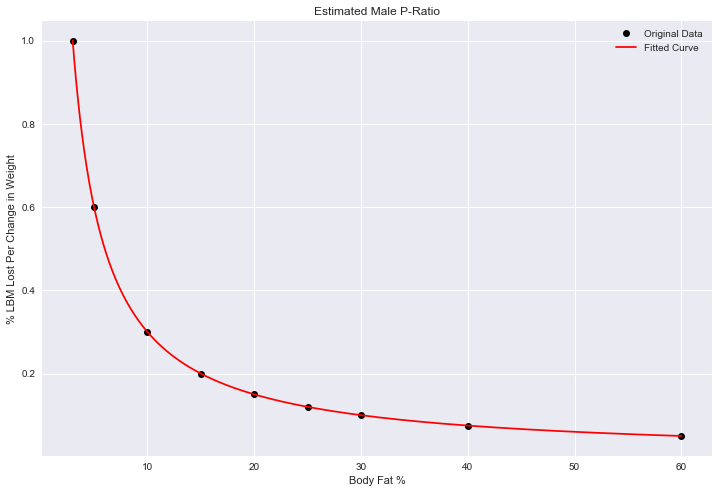# Using P-Ratio to Plan a Diet with Python and Excel

As a very goal oriented person, it helps me to define a structure and a finish line, especially when it comes to something as mentally difficult as dieting.

When we estimate how much weight we want to lose, we ballpark without concern to the muscle that will usually decrease along with it. Many people find they reach their “goal weight” and still don’t achieve the “toned” look, because they neglected to factor this in and adjust the goal accordingly.

The P-Ratio (or partitioning ratio), as I simplify it, defines what portion of weight loss is lean body mass as a function of current body fat (see Lyle McDonald’s article for a great overview and a more precise definition).

With the P-Ratio, given initial inputs for weight and body fat, you can calculate what weight you will reach your goal body fat at.

Because the P-Ratio is a function of body fat, and body fat is changing as we diet, we need a little calculus to help. With body fat as BF weight as W, fat as F, and lean body mass as L:

(1)(2)Taking the derivative of 2 using the quotient rule:Which we write as

(3)But from equation 1 we find

(4)Rearranging equations 1 and 4 we substituteUsing 1 and 2 we rearrange for L

(5)and plug in to the aboveMultiplying both sides by dW and canceling the W’s in the numeratorBut we’ve defined, how much LBM changes per change in weight, to be the P-Ratio, which is simply a function of BF. This lets us sum up the math neatly:

(6)## What is our P-Ratio function?

Ideally one could gather enough data to perform regression and find, but accurate body fat measurements are notoriously difficult to source (see a great meta analysis here), and beyond that, there is evidence that P-Ratio varies by individual (think of this variance as a modern take on endo-meso-ecto body types).

I initially threw together a few data points based on anecdotal reports and compared a few different models.Based on simplicity of model, I chose to use the linear model, where

(7)## Using Python to Optimize and Integrate

I entered the anecdotal P-Ratio values as below, with the female values shifted by 7% (approximating a man’s 10% being equivalent to a woman’s 17%, for example).

In :
import pandas as pd
import numpy as np
from scipy.optimize import curve_fit
import matplotlib.pyplot as plt
import seaborn as sns
sns.set()
%matplotlib inline
plt.rcParams["figure.figsize"] = 12,8

In :
defvals = np.array([[.60, 0.05],
[.40, 0.075],
[.30, 0.1],
[.25, 0.12],
[.20, 0.15],
[.15, 0.2],
[.10, 0.3],
[.05, 0.6],
[.03, 1]])

male = np.copy(defvals)

female = np.copy(defvals)
female[:, 0] += 0.07

maledf = pd.DataFrame(male, columns = ['Bodyfat', 'P-Ratio'])
femaledf = pd.DataFrame(female, columns = ['Bodyfat', 'P-Ratio'])

In :
print(maledf)

   Bodyfat  P-Ratio
0     0.60    0.050
1     0.40    0.075
2     0.30    0.100
3     0.25    0.120
4     0.20    0.150
5     0.15    0.200
6     0.10    0.300
7     0.05    0.600
8     0.03    1.000


These values can be interpreted best with an example: At 30% body fat (0.30), 10% of each pound lost will be lean body mass. This number increases as you become leaner, to the point that at 5% body fat, over half of each pound of weight lost will be muscle (60%).

In :
print(femaledf)

   Bodyfat  P-Ratio
0     0.67    0.050
1     0.47    0.075
2     0.37    0.100
3     0.32    0.120
4     0.27    0.150
5     0.22    0.200
6     0.17    0.300
7     0.12    0.600
8     0.10    1.000


I used SciPy’s curve_fit functionality to minimize least squares error and calculate the coefficients a and b given the P-ratio values input.

In :
def func(x, a, b): # our chosen P-ratio model
return 1/(a+b*x)

x=male[:,0] # body fat as input
y=male[:,1] # our model p-ratio as output

xf = female[:,0]
yf = female[:,1]

popt, pcov = curve_fit(func, x, y)
poptf, pcovf = curve_fit(func, xf, yf)

In :
print("Male Coefficients:", popt)
print("Female Coefficients:", poptf)

Male Coefficients: [  5.17506793e-09   3.33333332e+01]
Female Coefficients: [ -2.33333333  33.33333333]


To double check our figures, I plotted the predicted formula against our original data points

In :
plt.plot(x*100, y, 'ko', label="Original Data")
x_pred = np.linspace(x*100, x[-1]*100, 1000)
plt.plot(x_pred, func(x_pred/100, *popt), 'r-', label="Fitted Curve")
plt.xlabel("Body Fat %")
plt.ylabel("% LBM Lost Per Change in Weight")
plt.title("Estimated Male P-Ratio")
plt.legend()
plt.show()With those figures confirmed, we are ready to actually calculate our goal weight based on initial inputs! SymPy does a great job of integrating 6 for us and we solve for Goal Weight.

In :
import sympy
from fractions import Fraction

BF = sympy.Symbol("BF")
a, b = popt

# to fix polynomialdivisionerror in SymPy
a, b = float(Fraction(a).limit_denominator()), float(Fraction(b).limit_denominator())

a, b = sympy.sympify(a), sympy.sympify(b)

init_bodyfat = 20
init_weight = 160
final_bodyfat = 10

integral = sympy.integrate((1-BF-1/(a+b*BF))**(-1), (BF, round(init_bodyfat/100.0,2),
round(final_bodyfat/100.0,2)))
integral = np.float(integral)

final_weight = round(np.exp(integral + np.log(init_weight)),1)
print("With an initial weight and body fat of", init_weight, "lbs and",
init_bodyfat, "% body fat, you will need to reach",
final_weight, "lbs to hit", final_bodyfat, "% body fat.")


“With an initial weight and body fat of 160 lbs and 20 % body fat, you will need to reach 136.9 lbs to hit 10 % body fat.”

I included the above calculations with totally customizable starting parameters in my “fitness suite” that scrapes and uses your own data from popular fitness apps to deliver customized results.

In the future, I’d like to expand it into an app that can aggregate anonymized user data to calculate an empirical value for the P-ratio.

In the meantime, I put together this same material in an Excel spreadsheet for easy distribution and modification.